# 多层感知机与反向传播算法

23 三月 2021, 13:55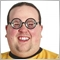0
685

### 介绍

• 这两种方法的普及性日益增加，因此在 Matlab、R、Python、C++ 等领域开发了大量的库，它们接收到一个训练集作为输入，并自动为问题创建合适的网络。
• 然而，当使用现成的库时，很难理解到底发生了什么以及我们如何得到一个优化的网络。对解决方案基础知识的理解对于这些方法的进一步发展至关重要。在本文中，我们将创建一个非常简单的神经网络结构。
• 让我们试着理解基本的神经网络类型是如何工作的（包括单神经元感知机和多层感知机）。我们将考虑一个令人兴奋的算法，它负责网络训练（梯度下降反向传播）。现有的复杂模型往往基于这样简单的网络模型。

### 历史概述

• 第一个神经网络是由沃伦·麦卡洛赫（Warren McCulloch）和沃尔特·皮特（Walter Pitts）于1943年提出的。他们写了一篇关于神经元应该如何工作的文章，他们还根据自己的想法建立了一个模型：他们用电路创建了一个简单的神经网络。
• 人工智能研究进展迅速，1980年，福岛邦彦（Kunihiko Fukushima）开发了第一个真正的多层神经网络。
• 神经网络的最初目的是创造一个计算机系统，能够以类似人脑的方式解决问题。然而，随着时间的推移，研究人员改变了研究重点，开始使用神经网络来解决各种特定任务。现在，神经网络执行各种各样的任务，包括计算机视觉、语音识别、机器翻译、社交媒体过滤、棋盘游戏或视频游戏、医疗诊断、天气预报、时间序列预测、图像/文本/语音识别等。

### 感知机

• z = sum(weight_i * x_i) + bias

• 如果 z >= 0.0, y = 1.0 否则就是 0.0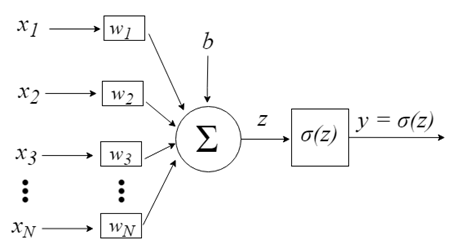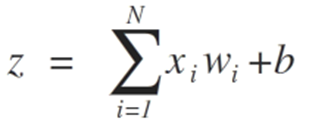b提供了更高的自由度，因此它不依赖于输入。通常这对应着 "偏离（bias）". 然后z值通过σ激活函数，该函数负责将该值限制在某个间隔内（例如，0-1），从而产生最终输出和神经元值。一些使用的激活函数包括阶跃(step)、S形(sigmoid)、双曲正切、softmax 和 ReLU（“校正线性单元”）。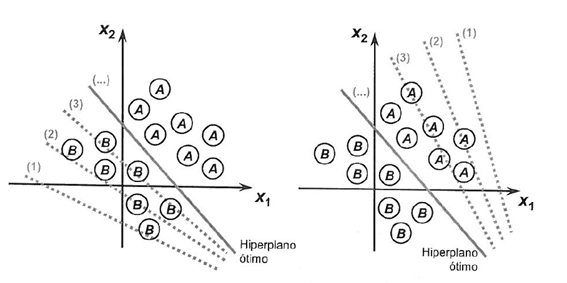### 随机梯度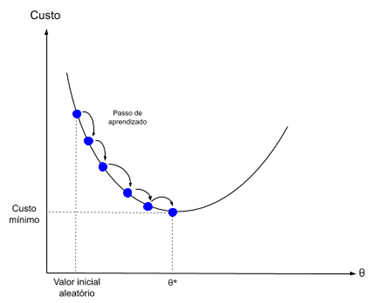• w = w + learning_rate * (expected - predicted) * x

• 学习率（Learning rate）：用于限制每次更新时每个权重的修正量。
• 历元（Epochs）-更新权重时，需要运行多少次训练数据。

1. 为每个 epoch 循环.

2. 在 epoch 中为训练数据的每一行循环.

3. 循环每个权重，一次更新一行。

• w(t+1)= w(t) + learning_rate * (expected(t) - predicted(t)) * x(t)

• bias(t+1) = bias(t) + learning_rate * (expected(t) - predicted(t)).

1. 做预测

2. 优化网络权重

`#define nINPUT 3`

1. 进行预测

```// Make a prediction with weights
template <typename Array>
double predict(const Array &X[][nINPUT], const Array &weights[], const int row=0)
{
double z = weights;
for(int i=0; i<ArrayRange(X, 1)-1; i++)
{
z+=weights[i+1]*X[row][i];
}
return activation(z);
}
```

#### 神经元传输：

```//+------------------------------------------------------------------+
//|                Transfer neuron activation                        |
//+------------------------------------------------------------------+
double activation(const double activation) //#
{
return activation>=0.0?1.0:0.0;
}
```

`double weights[] = {-0.1, 0.20653640140000007, -0.23418117710000003};`

```#define nINPUT 3
//+------------------------------------------------------------------+
//| Script program start function                                    |
//+------------------------------------------------------------------+
void OnStart()
{
//---
random.seed(42);
double dataset[][nINPUT] = {     //X1     //X2   //Y
{2.7810836,2.550537003,0},
{1.465489372,2.362125076,0},
{3.396561688,4.400293529,0},
{1.38807019,1.850220317,0},
{3.06407232,3.005305973,0},
{7.627531214,2.759262235,1},
{5.332441248,2.088626775,1},
{6.922596716,1.77106367,1},
{8.675418651,-0.242068655,1},
{7.673756466,3.508563011,1}
};
double weights[] = {-0.1, 0.20653640140000007, -0.23418117710000003};
for(int row=0; row<ArrayRange(dataset, 0); row++)
{
double predict = predict(dataset, weights, row);
printf("Expected=%.1f, Predicted=%.1f", dataset[row][nINPUT-1], predict);
}
}
//+------------------------------------------------------------------+
// Make a prediction with weights
template <typename Array>
double predict(const Array &X[][nINPUT], const Array &weights[], const int row=0)
{
double z = weights;
for(int i=0; i<ArrayRange(X, 1)-1; i++)
{
z+=weights[i+1]*X[row][i];
}
return activation(z);
}
//+------------------------------------------------------------------+
//|                Transfer neuron activation                        |
//+------------------------------------------------------------------+
double activation(const double activation) //#
{
return activation>=0.0?1.0:0.0;
}
```

activation = (w1 * X1) + (w2 * X2) + b

activation = (0.206 * X1) + (-0.234 * X2) + -0.1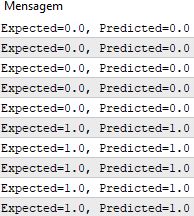2. 优化网络权重

``` //+------------------------------------------------------------------+
//|  Estimate Perceptron weights using stochastic gradient descent   |
//+------------------------------------------------------------------+
template <typename Array>
void train_weights(Array &weights[], const Array &X[][nINPUT], double l_rate=0.1, int n_epoch=5)
{
ArrayResize(weights, ArrayRange(X, 1));
for(int i=0; i<ArrayRange(X, 1); i++)
{
weights[i]=random.random();
}

for(int epoch=0; epoch<n_epoch; epoch++)
{
double sum_error = 0.0;
for(int row=0; row<ArrayRange(X, 0); row++)
{
double y = predict(X, weights, row);
double error = X[row][nINPUT-1] - y;
sum_error += pow(error, 2);
weights = weights + l_rate * error;

for(int i=0; i<ArrayRange(X, 1)-1; i++)
{
weights[i+1] = weights[i+1] + l_rate * error * X[row][i];
}
}
printf(">epoch=%d, lrate=%.3f, error=%.3f",epoch, l_rate, sum_error);
}
}
```

```#define nINPUT 3
//+------------------------------------------------------------------+
//| Script program start function                                    |
//+------------------------------------------------------------------+
void OnStart()
{
//---
random.seed(42);
double dataset[][nINPUT] = {     //X1     //X2   //Y
{2.7810836,2.550537003,0},
{1.465489372,2.362125076,0},
{3.396561688,4.400293529,0},
{1.38807019,1.850220317,0},
{3.06407232,3.005305973,0},
{7.627531214,2.759262235,1},
{5.332441248,2.088626775,1},
{6.922596716,1.77106367,1},
{8.675418651,-0.242068655,1},
{7.673756466,3.508563011,1}
};
double weights[];
train_weights(weights, dataset);
ArrayPrint(weights, 20);
for(int row=0; row<ArrayRange(dataset, 0); row++)
{
double predict = predict(dataset, weights, row);
printf("Expected=%.1f, Predicted=%.1f", dataset[row][nINPUT-1], predict);
}
}
//+------------------------------------------------------------------+
// Make a prediction with weights
template <typename Array>
double predict(const Array &X[][nINPUT], const Array &weights[], const int row=0)
{
double z = weights;
for(int i=0; i<ArrayRange(X, 1)-1; i++)
{
z+=weights[i+1]*X[row][i];
}
return activation(z);
}
//+------------------------------------------------------------------+
//|                Transfer neuron activation                        |
//+------------------------------------------------------------------+
double activation(const double activation) //#
{
return activation>=0.0?1.0:0.0;
}
//+------------------------------------------------------------------+
//|  Estimate Perceptron weights using stochastic gradient descent   |
//+------------------------------------------------------------------+
template <typename Array>
void train_weights(Array &weights[], const Array &X[][nINPUT], double l_rate=0.1, int n_epoch=5)
{
ArrayResize(weights, ArrayRange(X, 1));
ArrayInitialize(weights, 0);

for(int epoch=0; epoch<n_epoch; epoch++)
{
double sum_error = 0.0;
for(int row=0; row<ArrayRange(X, 0); row++)
{
double y = predict(X, weights, row);
double error = X[row][nINPUT-1] - y;
sum_error += pow(error, 2);
weights = weights + l_rate * error;

for(int i=0; i<ArrayRange(X, 1)-1; i++)
{
weights[i+1] = weights[i+1] + l_rate * error * X[row][i];
}
}
printf(">epoch=%d, lrate=%.3f, error=%.3f",epoch, l_rate, sum_error);
}
}
```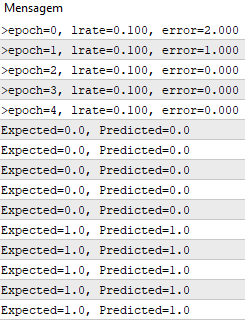### 多层感知机

• 将神经元组合成层

一个神经元能做的事情不多。但是神经元可以组合成一个多层结构，每一层有不同数量的神经元，并形成一个称为多层感知器（Multi-Layer Perceptron, MLP）的神经网络。输入向量 X 通过初始层传入，该层的输出值被输入到下一层，以此类推，直到最后一层输出最终结果。这个网络可以被组织成几个层次，使得它很深，能够学习越来越复杂的关系。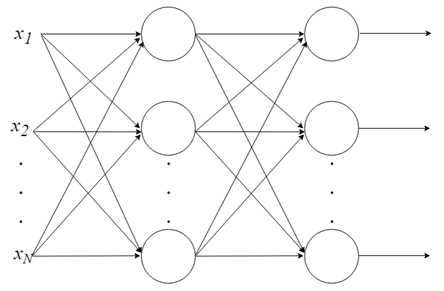### MLP 训练

在网络开始工作之前，需要对该网络进行训练。就像教孩子读书一样，在机器学习的背景下，监督训练被用来训练MLP，但是它是如何工作的呢？

• 我们有一组带标签的数据，我们已经知道哪个是正确的输出。学习是建立在输入和输出之间有联系的思想基础上的。
• 监督学习问题又分为“回归”问题和“分类”问题。在回归问题中，我们试图预测连续输出的结果，这意味着我们试图将输入变量映射到某个连续函数。在分类问题中，我们试图以离散输出预测结果。换句话说，我们尝试将输入变量映射到不同的类别中。

• 根据房地产市场的房屋大小数据，尝试预测其价格。价格取决于大小是一个连续的结果，所以这是一个回归问题。
• 这个问题可以转化为一个分类问题——预测“房子的售价是否能超过规定的价格”。这里的房子将按价格分为两类。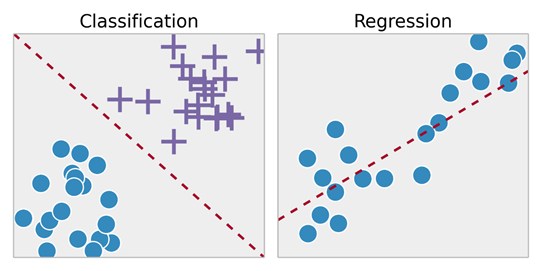### 反向传播

1. 前向传递：输入通过网络并接收输出预测（此步骤也称为传播步骤）。

2. 反向传递：在网络的最后一层（预测层）计算损失函数梯度。然后使用它递归应用链式规则来更新网络中的权重（也称为权重更新或反向传播）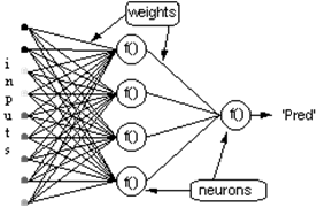(1)

= E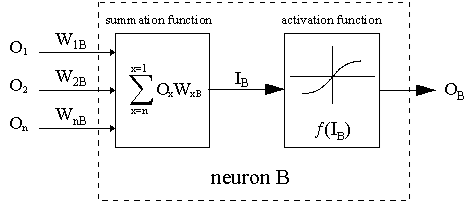(2)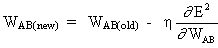where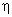- 是学习率参数，并且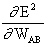(3)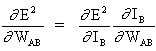(4)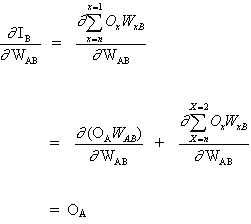(5)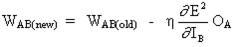1. B 是一个输出神经元;

2. B 是一个隐藏神经元.

(6)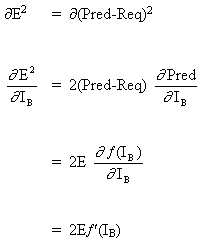(7)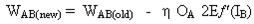(8)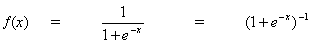(9)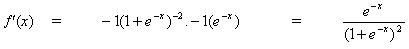(10)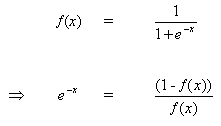(11)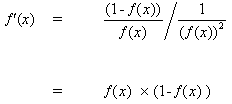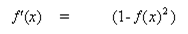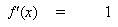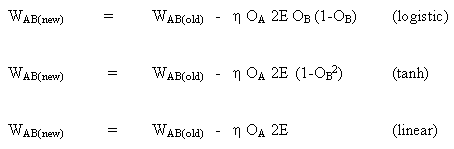B 是一个隐藏神经元

(12)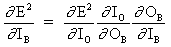(13)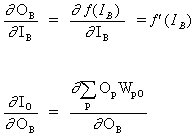(14)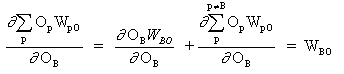(15)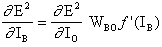(16)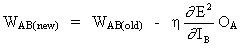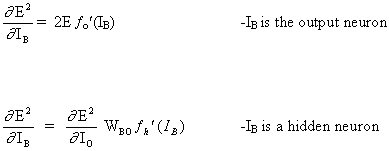### 示例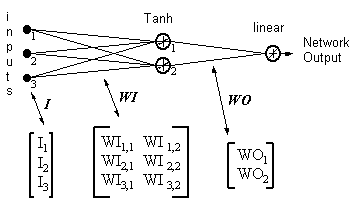HID = [Tanh(I T.WI)] T- 隐藏神经元的输出

ERROR = (网络输出 - 所需输出)

LR = 学习率

(17)

WO = WO - ( LR x ERROR x HID )

(18)

WI = WI - { LR x [ERROR x WO x (1- HID 2)] . I T } T

“反向传播家庭相册”-技术报告C/TR96-05，澳大利亚新南威尔士州麦格理大学计算系.

### MLP模型的应用

1.       网络的初始化.

2.       前馈.

3.       反向传播.

4.       网络的训练.

5.       预测.

1. 网络初始化

```double random(void)
{
return ((double)rand())/(double)SHORT_MAX;
}
```

```// Forward propagate input to a network output
void forward_propagate(void)
{
//calculate the outputs of the hidden neurons
//the hidden neurons are tanh
int i = 0;
for(i = 0; i<numHidden; i++)
{
hiddenVal[i] = 0.0;
for(int j = 0; j<numInputs; j++)
{
hiddenVal[i] += (X[patNum][j] * weightsIH[j][i]);
}
hiddenVal[i] = tanh(hiddenVal[i]);
}
//calculate the output of the network
//the output neuron is linear
outPred = 0.0;
for(i = 0; i<numHidden; i++)
{
outPred += hiddenVal[i] * weightsHO[i];
}
//calculate the error
errThisPat = outPred - y[patNum];
}
```

3. 反向传播

```//+------------------------------------------------------------------+
//|        Backpropagate error and change network weights            |
//+------------------------------------------------------------------+
void backward_propagate_error(void)
{
//adjust the weights hidden-output
for(int k = 0; k<numHidden; k++)
{
double weightChange = LR_HO * errThisPat * hiddenVal[k];
weightsHO[k] -= weightChange;
//regularisation on the output weights
regularisationWeights(weightsHO[k]);
}
// adjust the weights input-hidden
for(int i = 0; i<numHidden; i++)
{
for(int k = 0; k<numInputs; k++)
{
double x = 1 - pow(hiddenVal[i],2);
x = x * weightsHO[i] * errThisPat * LR_IH;
x = x * X[patNum][k];
double weightChange = x;
weightsIH[k][i] -= weightChange;
}
}
}
```

regularizationWeights 方法仅用于正则化介于-5和5之间的权重。

```//regularisation on the output weights
void regularisationWeights(double &weight)
{
weight<-5?weight=-5:weight>5?weight=5:weight=weight;
}
```

4. 网络的训练

```//# Train a network for a fixed number of epochs
void train(void)
{
for(int j = 0; j <= numEpochs; j++)
{
for(int i = 0; i<numPatterns; i++)
{
//select a pattern at random
patNum = rand()%numPatterns;
//calculate the current network output
//and error for this pattern
forward_propagate();
backward_propagate_error();
}
//display the overall network error
//after each epoch
calcOverallError();
printf("epoch = %d RMS Error = %f",j,RMSerror);
}
}
```

5. 预测

```// # Make a prediction with a network
void predict(void)
{
for(int i = 0; i<numPatterns; i++)
{
patNum = i;
forward_propagate();
printf("real = %d predict = %f",y[patNum],outPred);
}
}
```

### 结论

MLP_Script.mq5 (6.98 KB)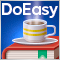DoEasy 函数库中的时间序列（第六十一部分）：品种即时报价序列集合DoEasy 函数库中的时间序列（第六十部分）：品种即时报价数据的序列列表DoEasy 函数库中的价格（第六十二部分）：实时更新即时报价序列，为操控市场深度做准备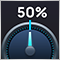自适应算法(第三部分): 放弃优化